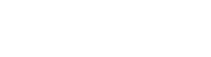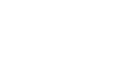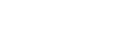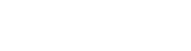## MATHEMATICS PAPER 1 - 2019 KCSE KASSU JOINT MOCK EXAMS (QUESTIONS AND ANSWERS)

Instructions to candidates

• The paper contains TWO sections: Section I and Section II.
• Answer ALL the questions in section I and strictly any five questions from Section II.
• Marks may be given for correct working even if the answer is wrong.
• Non-programmable silent electronic calculators and KNEC mathematical tables may be used, except where stated otherwise.

SECTION I (50 MARKS)
Attempt all the questions in the spaces provided

1. Evaluate:    (3 marks)1. Use logarithms, correct to 4 decimal places, to evaluate    (4mks)2. Given that sin (x+30)°=-0.7660, find x, to the nearest degree, for 0°≤x≤360° (3mks)
3. Simplify: (3 marks)4. The interior angle of a polygon is eleven times the exterior angle. Find the sum of the interior angles of the polygon.        (3mks)
5. A line L1 passes through point (2, 3) and has a gradient of 3. Another line L2 is perpendicular to L1 and meets it at a point where y=6. Find the equation of L2 in the form ax+by=c where a, b and c are integers.     (3 marks)
6. Solve the following inequalities and represent the solution on a single number line.     (3mks)
3(x-2)<27x≤81(1/2x+2)
1. From the top of a building which is 15m above the ground, the angle of depression of an object on the ground is 30o and the angle of elevation of an aircraft vertically above the object is 48o. Calculate the height of the aircraft above the object.        (4 mks)
1. A train moving at 120km/h approaches a bridge 50M long which is 120M away. If the train takes 9secs to completely cross the bridge, determine the length of the train in metres.(3mks)
1. The diagram below is point of a figure which has rotational symmetry of order 4 about O.Complete the figure and draw all the lines of symmetry of the completed figure (use dotted lines of symmetry).    (3 marks)
1. Find the equation of the image of the line whose gradient is 3 and passes through the point (2, 1) under a translation by [ 3-2 ] in the form y=mx+c(3 marks)
2. A Kenyan company received 90,000 US dollars (\$) which they converted into Kenya Shillings at the rates below:-
1 \$                       84.25                                                85.75
1 £                       104.15                                             105.50
1. Calculate the amount received in Kshs                                          (2 marks)
2. The company used the money to buy a car. The money was therefore converted into sterling pound (£) and the car purchased from Britain at 10% discount. Determine the price of the car to the nearest sterling pound.   (2 marks)
3. Solve for y in the equation 32(y-3)×8(y+4)=64÷2y(3 marks)
4. Find the acute angle x, given that cos x=sin 2x(2 marks)
5. In the figure below, triangle DAC is similar to triangle ABC. If AB=9cm and CD=4cm, find AC(3mks)6. Mary is four times as old as her daughter. In seven years time, the sum of their ages will be 59 years. Determine their ages three years ago.   (3 marks)

SECTION II (50MKS)
ANSWER ANY FIVE QUESTIONS FROM THIS SECTION IN THE SPACES PROVIDED

1. From a reservoir, water flows through a cylindrical pipe of diameter 0.2M at a rate of 0.35M/s. (Take π=22/7)
1. Determine the number of litres of water discharged from the reservoir in one hour.          (4mks)
2. The water flows from the reservoir for 18hours per day for 25days per month and serves a population of 2500 families. Determine the average consumption of water per family per month giving your answer to the nearest 100 litres.    (4mks)
3. The water is charged at the rate of sh. 4.50 per 100 litres. Calculate the average water bill per family per month.   (2mk)
1. A solid is partly a cone and partly a hemisphere. The radius of the hemisphere is 5cm and the height of the solid is 17cm as shown in the figure below.1. Determine the volume of the:-
1. conical part   (2marks)
2. hemispherical part      (2marks)
3. whole solid    (2marks)
2.  Calculate the surface area of the solid.  (4marks)
1. Two matrices A and B are such that.
1. Given that the determinant of AB=4, find K
2. Rose bought 200 bags of sugar and 300 bags of rice for a total of sh. 850000. Jane bought 90 bags of sugar and 120 bags of rice for a total of sh. 360000. If the price of a bag of sugar is sh. X and that of a bag of rice is sh. Y
3.
1. Find by matrix method, the price of one bag of each item.  (4mks)
2. Kali bought 225 bags of sugar and 360 bags of rice. He was given a total discount of sh. 33300. If the discount on the price of a bag of rice was 2%, calculate percentage discount on the price of  a bag of sugar.          (2mks)
1.
1. Using a ruler and a pair of compass only, construct a rhombus PQRS such that PQ=6cm and <PQR=135o    (3 marks)
2. Drop a perpendicular from R to meet PQ extended at N. Measure QN.       (2 marks)
3. Bisect <PQR and <SPQ and let the two bisectors meet at M. Measure MP (3marks)
4. Determine the area of triangle PQM.      (2marks)
2.
1. On the grid provided, draw the square whose vertices are. A(6, -2), B(7, -2), C(7, -1) and     D(6,-1).   (1mk)
2. On the same grid,
1. Draw  A’B’C’D’ the image of ABCD, under the enlargement scale factor 3, center (9, -4)            (3mks)
2. Draw A”B”C”D” the image of A’B’C’D’ under a reflection in the line X=0                                     (2mks)
3. Draw A’’’B’’’C’’’D’’’ the image of A”B”C”D” under a rotation of +90° about(0, 0)            (2mks)
3. Describe a single transformation that maps A’B’C’D’ onto A’’’B’’’C’’’D’’’ (2mks)
3. In the figure below, AD is a diameter of a circle center O. Angle FAD=64o, angle EFD=30o, angle ADB=50o and the length AD=7cm.1. Giving reasons, determine the size of the angles:
1. FED     (2 marks)
2. DCB      (3 marks)
3. Reflex EOB   (2 marks)
2. Determine the area of the shaded region.  (3 marks)
1. The equation of a curve is y=6x2-12x-18
1. Find;
1. the x-intercepts of the curve.     (3mks)
2. the y- intercept of the curve     (1mk)
2.
1. Determine the stationary points of the curve     (3mks)
2. For the points in b (i) above, determine whether it is a minimum or a maximum point      (1mk)
3. Sketch the curve.       (2mks)
1. Four towns R, T, K and G are such that T is 84km directly to the North of R and K is on a bearing of 295o from R at a distance of 60km. G is on a bearing of 340o from K and a distance of 30km. Using a scale of 1cm to represent 10km, make an accurate scale drawing to show the relative positions of the towns.  (6 marks)
1. Use your diagram to find:
1. distance and bearing of T from K.     (2 marks)
2. distance and bearing of G from T.      (2 marks)## MARKING SCHEME• ✔ To read offline at any time.
• ✔ To Print at your convenience
• ✔ Share Easily with Friends / Students